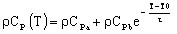# Volumetric heat capacity, exponential function of T

## Presentation

This model defines a volumetric heat capacity ρCp as an exponential function of the temperature for an isotropic or anisotropic material.

## Mathematical model

The volumetric heat capacity is an exponential function of the temperature.

The corresponding mathematical formula is written:where:

• T0 is the reference temperature (degree)
• ρCpa + ρCpb is the volumetric heat capacity at T0 (J/m3/degree)
• ρCpa is a constant quantity (J/m3/degree)
• τ is the temperature constant (positive or negative) (degree)

The shape of ρCp(T) curve is presented in the figure below.